Regular Expressions

# Regular Expressions - Theory of Computation - Computer Science Engineering (CSE)

Part V. Regular Expressions
We found in earlier sections that a language can be described by using an NFA or DFA. But a more concise way of describing a lnguage is by using regular expressions. Thus one way of describing a language is via the notion of regular expressions.
For example,
ab,
a,
ab,
(ab)+,
abcd,
x/y,
a/bcd,
letter(letter/digit)*,
a(b/c)d*
are different examples of regular expressions. We will learn the meaning of all these symbols later. A regular expression generates a set of strings.
For example, the regular expression, a∗ generates the strings, ε, a, aa, aaa, aaaa.....
The regular expression, a(b/a) generates the strings ab, ba.

7 Definition of a Regular Expression
Let ∑ denotes the character set, ie. a, b, c....z.
The regular expression is defined by the following rules:

Rule 1: Every character of ∑ is a regular expression.
For example, a is a regular expression, b is a regular expression, c is a regular expression and so on.

Rule 2: Null string, ε is a regular expression.

Rule 3: If R1 and R2 are regular expressions, then R1 R2 is also a regular expression.
For example, let R1 denotes abc and

let R2 denotes (pq)*,
then abc(pq)* is also a regular expression.

Rule 4: If R1 and R2 are regular expressions, then R1 / R2 is also a regular expression.
For example, let R1 denotes (ab)and
let R2 denotes cd,
then (ab)+/(cd) is also a regular expression.

Rule 5: If R is a regular expression, then (R) is also a regular expression.
For example, let R denotes adc,
then (adc) is also a regular expression.

Rule 6: If R is a regular expression, then R*is also a regular expression.
For example, let R denotes ab,
then (ab)*is also a regular expression.

Rule 7: If R is a regular expression, then R+ is also a regular expression.
For example, let R denotes pqr,
then (pqr)+is also a regular expression.

Rule 8: Any combination of the preceding rules is also a regular expression.
For example, let R1 denotes (ab)*,
let R2 denotes d+,
let R3 denotes pq,
then ((ab)*/(d+pq)*)* is also a regular expression.

Strings Generated from a Regular Expression
A regular expression generates a set of strings.
1. A regular expression, a* means zero or more repretitions of a.
So the strings generated from this regular expression are,
ε,
a,
aa,
aaa,
aaaa, and so on.

2. A regular expression, pmeans one or more repretitions of a.
So the strings generated from this regular expression are,
p,
pp,
ppp,
pppp, and so on.

3. A regular expression, a/b means the string can be either a or b.
So the strings generated from this regular expression are,
a,
b.

4. A regular expression, (a/b)c means a string that starts with either a or b and will be followed by c.
So the strings generated from this regular expression are,
ac,
bc

5. A regular expression, (a/b)c(d/e) means that a string will begin with either a or b, followed by c, and will end with either d or e.
So the strings generated from this regular expression are,
acd,
ace,
bcd,
bce.

Following shows some examples for the strings generated from regular expressions:
Regular Expression Strings Generated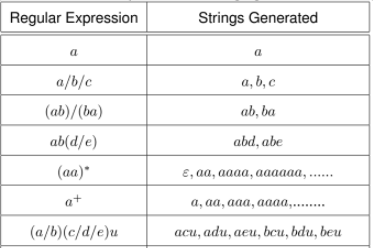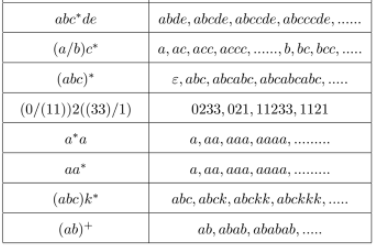Example 1:
Write regular expression for the language,
L={aa, aaaa, aaaaaa, aaaaaaaa, ......}
Here aa repeats a number of times starting from 1. So the regular expression is,
(aa)+

Example 2:
Write regular expression for the language,
L={0, 1, 2}
The regular expression is,
0/1/2

Example 3:
Write regular expression for the language, L over {0,1}, such that every string in L begins with 00 and ends with 11.
The regular expression is,
00(0/1)*11.

Example 4:
Write regular expression for the language, L over {0,1}, such that every string in L contains alternating 0s and 1s.
The regular expression is,
(0(10)*)/(0(10)*1)/(1(01)*)/(1(01)*0)

Example 5:
Write regular expression for the language, L over {0,1}, such that every string in L ends with 11.
The regular expression is,
(0/1)*11

Example 6:
Write regular expression for the language, L over {a,b,c}, such that every string in L contains a substring ccc.
The regular expression is,
(a/b/c)*ccc(a/b/c)*

Example 7:
Write regular expression for the language, L = {anbm|n ≥ 4, m ≤ 3}
This means that language contains the set of strings such that every string starts with at least 4 a’s and end with at most 3 b’s.
The regular expression is,
a4a*(ε/b/b2/b3)

Example 8:
Write regular expression for the language, L = {anbm|(n+m) is even }
n+m can be even in two cases.
Case 1: Both n and m are even,
The regular expression is,
(aa)(bb)*

Case 2: Both n and m are odd,
The regular expression is,
(aa)a(bb)*b
Then the regular expression for L is,
((aa)*(bb)*)/((aa)*a(bb)*b)

8 Transforming Regular Expressions to Finite Automata
For every regular expression, a corresponding finite automata exist.
Here we will learn how to construct a finite automation corresponding to a regular expression.
1. The NFA corresponding to regular expression, ε is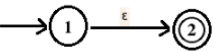2. The NFA corresponding to regular expression, a is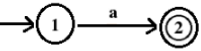3. The NFA corresponding to regular expression, a | b is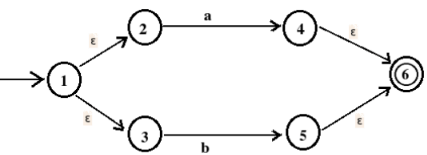4. The NFA corresponding to regular expression, ab is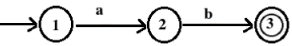5. The NFA corresponding to regular expression, a* is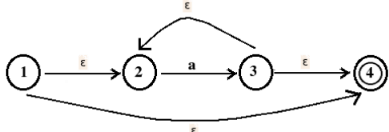Example 1:
Construct a finite automation for the regular expression, ab*c.
The finite automation corresponding to b* is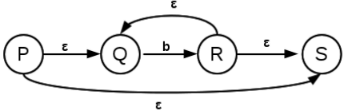Then the finite automation corresponding to ab*c is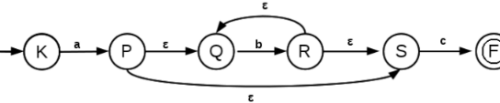Example 2:
Construct a finite automation for the regular expression, (ab)*.
The finite automation corresponding to ab is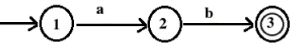The finite automation corresponding to (ab)* is,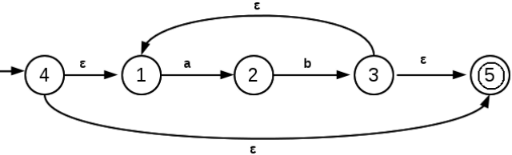Example 3:
Find the NFA corresponding to regular expression (a|b)*a.
The NFA for a|b is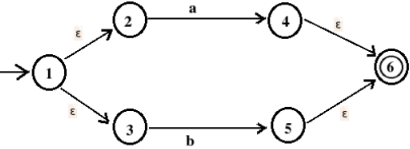The NFA corresponding to (a|b)* is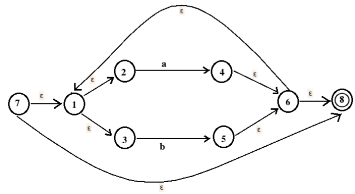The NFA corresponding to (a|b)*a is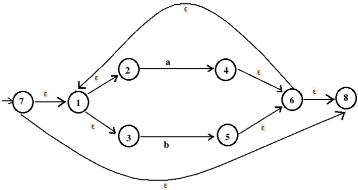9 DFA to Regular Expression Conversion

Note: In this section, we use + symbol instead of / operator. For example, a/b is written as a+b. We can find out the regular expression for every deterministic finite automata (DFA).

Arden’s Theorem
This theorem is used to find the regular expression for the given DFA.
Let P and Q are two regular expressions,
then the equation, R = Q + RP has the solution,
R = QP*.

Proof:
Q + RP = Q + (QP*)P,
since R = QP*.
= Q(ε + P*P)
= Q[ε/(P*P)]
= QP*
= R

Thus if we are given, R = Q + RP , we may write it as,
R = QP*
The process is demonstrated using the following examples:

Example 1:
Find the regular expression corresponding to the following DFA,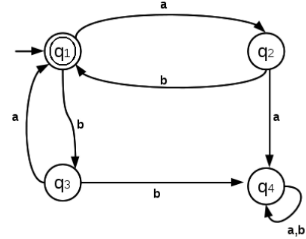Here we write equations for every state.
We write,
q1 = q2b+ q3a + ε
The term q2b because there is an arrow from q2 to q1on input symbol b.
The term q3a because there is an arrow from q3 to q1on input symbol a.
The term ε because qis the start state.
q2 = q1a
The term q1a because there is an arrow from q1 to q2on input symbol a.
q3 = q1b
The term q1b because there is an arrow from q1 to q3on input symbol b.
q4 = q2a + q3b + q4a + q4b
The term q2a because there is an arrow from q2 to q4on input symbol a.
The term q3b because there is an arrow from q3 to q4on input symbol b.
The term q4b because there is an arrow from q4 to q4on input symbol b.

The final state is q1.
Putting q2 and q3 in the first equation (corresponding to the final state), we get,
q1 = q1ab + q1ba + ε
q1 = q1(ab + ba) + ε
q1 = ε + q1(ab + ba)
From Arden’s theorem,
q= ε(ab + ba)*
q= (ab + ba)*

So the regular expression is, ((ab)/(ba))*

Example 2;
Find the regular expression corresponding to the following DFA,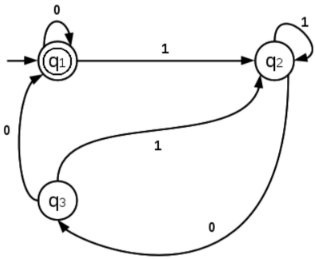Let us write the equations
q1 = q10 + q30 + ε
q2 = q11 + q21 + q31
q3 = q20

q2 = q11 + q21 + q31
q2 = q11 + q21 + (q20)1
q2 = q11 + q2(1 + 01)
q2 = q11(1 + 01)*(From Arden’s theorem)

Consider the equation corresponding to final state,
q1 = q10 + q30 + ε
q1 = q10 + (q20)0 + ε
q1 = q10 + (q11(1 + 01)*)0)0 + ε
q1 = q1(0 + 1(1 + 01)*)00) + ε
q1 = ε + q1(0 + 1(1 + 01)*)00)
q1 = ε(0 + 1(1 + 01)*)00)*
q1 = (0 + 1(1 + 01)*)00)*

Since q1 is a final state, the regular expression is,
(0/(1(1 + 01)*)00))*

Example 3:
Find the regular expression corresponding to the following DFA,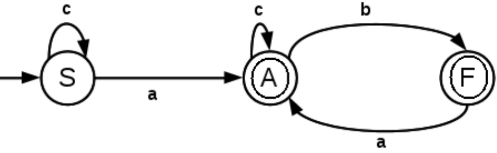The equations are,
S = Sc + ε
A = Ac + F a + Sa
F = Ab

S = Sc + ε
S = ε + Sc
S = εc*
S = c*

A = Ac + F a + Sa
A = Ac + F a + c*a
A = Ac + Aba + c*a
A = A(c + ba) + c*a
A = c*a + A(c + ba)
A = c*a(c + ba)*

F = Ab
F = (c*a(c + ba)*)b

There are two final states in the DFA, they are A and F.
The regular expression corrresponding to A is c*a(c + ba)*
The regular expression corrresponding to F is (c*a(c + ba)*)b

Then the regular expression for the DFA is
c*a(c + ba)∗ + (c*a(c + ba)*)b
That is, [c*a(c/(ba))*]/[(c*a(c/(ba))*)b]

Exercises:
1. Find the regular expression corresponding to the following DFA,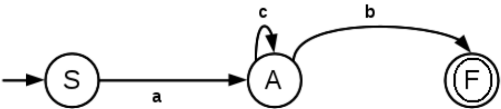2. Find the regular expression corresponding to the following DFA,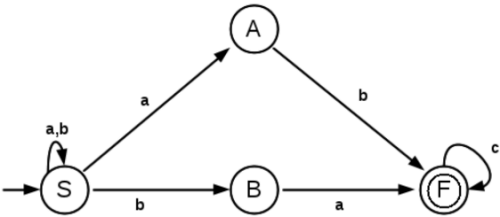3. Find the regular expression corresponding to the following DFA,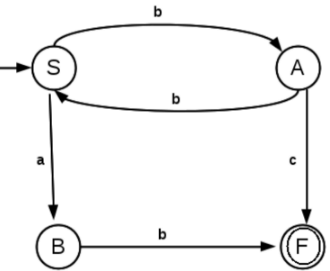4. Find the regular expression corresponding to the following DFA,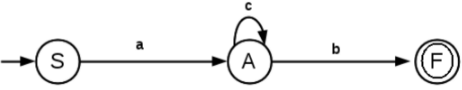5. Find the regular expression corresponding to the following DFA,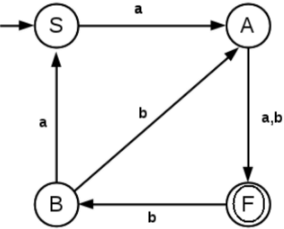6. Find the regular expression corresponding to the following DFA,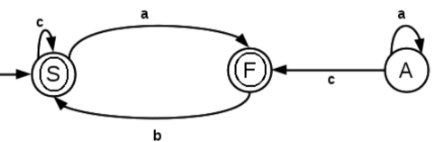The document Regular Expressions | Theory of Computation - Computer Science Engineering (CSE) is a part of the Computer Science Engineering (CSE) Course Theory of Computation.
All you need of Computer Science Engineering (CSE) at this link: Computer Science Engineering (CSE)

## Theory of Computation

18 videos|56 docs|44 tests

## FAQs on Regular Expressions - Theory of Computation - Computer Science Engineering (CSE)

 1. What is a regular expression?A regular expression is a sequence of characters that defines a search pattern. It is used to match and manipulate text based on certain patterns. In computer science, regular expressions are often used for tasks such as pattern matching, string searching, and data validation.
 2. What are the benefits of using regular expressions in computer science engineering?Regular expressions offer several benefits in computer science engineering. They provide a concise and powerful way to search, manipulate, and validate text. By using regular expressions, engineers can efficiently handle complex string operations, such as pattern matching, data extraction, and text substitution. Regular expressions also enable automation and efficiency in tasks like parsing and data validation.
 3. What are some common metacharacters used in regular expressions?Regular expressions use metacharacters to define patterns. Some commonly used metacharacters include: - "." (dot): Matches any single character except a newline. - "*" (asterisk): Matches zero or more occurrences of the preceding character. - "+" (plus): Matches one or more occurrences of the preceding character. - "?" (question mark): Matches zero or one occurrence of the preceding character. - "[ ]" (square brackets): Defines a character class and matches any character within the brackets.
 4. How can regular expressions be used for data validation in computer science engineering?Regular expressions are widely used for data validation tasks in computer science engineering. They provide a flexible and efficient way to ensure that input data matches a specific pattern or format. For example, engineers can use regular expressions to validate email addresses, phone numbers, credit card numbers, or any other structured data. By defining the desired pattern using regular expressions, engineers can easily check if the input data adheres to the expected format.
 5. Can regular expressions be used in programming languages other than CSE?Yes, regular expressions are widely supported in various programming languages and frameworks. They are not limited to computer science engineering and can be used in fields such as web development, data analysis, and system administration. Popular programming languages like Python, Java, JavaScript, and Ruby have built-in support for regular expressions. These languages provide libraries and functions that allow developers to easily work with regular expressions and perform various text manipulation tasks.

## Theory of Computation

18 videos|56 docs|44 testsExplore Courses for Computer Science Engineering (CSE) examSignup to see your scores go up within 7 days! Learn & Practice with 1000+ FREE Notes, Videos & Tests.
10M+ students study on EduRev
Track your progress, build streaks, highlight & save important lessons and more!
Related Searches

,

,

,

,

,

,

,

,

,

,

,

,

,

,

,

,

,

,

,

,

,

;